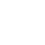Hello! Welcome to the website of Dongguan Youchen Electronic Technology Co., Ltd.

Youchen Technology

Chip Capacitor/Electrolytic Capacitor/Ceramic Capacitor Supplier
18929295505
Current Page: Home > News > Corporate news
News

#### Method for Identifying the Resistance Value of Chip Resistors

##### 2021-10-07

Chip capacitor resistorThe size of the value is usually related to temperature, material, length, and cross-sectional area. The physical quantity used to measure the resistance affected by temperature is the temperature coefficient, which is defined as the percentage of change in the resistance value for every 1 ℃ increase. The counting method for resistance value is as follows: on the commonly used surface of SMD resistors, it is represented in the form of letters or resistance values.

The first and second numbers represent the real number of the resistance.

If the number starting from the third digit is 0, it represents several tens of Ω (between 10 and 99 Ω). Column: 100 represents a resistance of 10 Ω, and 990 represents a resistance of 99 Ω.

3. If the number starting from the third digit is 1, it represents several hundred Ω (between 100 and 999 Ω). For example, 101 represents 100 Ω, 151 represents 150 Ω, and 951 represents 950 Ω.

If the number starting from the third digit is 2, it represents several thousand Ω (between 1000 and 9999 Ω). For example, 102 is 1K, 152 is 1.5K, and 992 is 9.9K.

If the number starting from the third digit is 3, it represents several tens of K (between 10K and 99K). For example, 103 represents 10K, 223 represents 22K, and 993 represents 99K.

If the number starting from the third digit is 4, it represents several hundred K (between 100K and 999K). For example, 104 represents 100K, 204 represents 200K, and 854 represents 850K.

7. If the number starting from the third digit is 5, it represents a few M (between 1M and 9.9). For example, 105 is 1M, 155 is 1.5M955 is 9.5M.

8. If the number starting from the third digit is 6, it represents ten M (between 100K and 999K). For example, 106 represents 10M566 represents 56M.

For the notation of four numbers, the first three digits are real numbers and the fourth digit is a multiple. 1001 is 1K, 1002 is 10K, and 1005 is 10M.Calibration method for resistor resistance value

1. Direct marking method: The resistance value on the surface of the resistor is marked with numbers and unit symbols, and its allowable error is directly expressed as a percentage. If there is no deviation on the resistor, the allowable error is ± 20%.

2. Character symbol method: Arabic numerals and character symbols are regularly combined to represent the nominal resistance value, and the allowable deviation is also represented by character symbols. The first digit represents the integer resistance value, while the last digit represents the first decimal resistance value and the second decimal resistance value. Text symbols, indicating allowable errors: DFGJKM allowable deviations are: ± 0.5% ± 1% ± 2% ± 5% ± 10% ± 20%

3. Number method: A method of marking the rated value on a resistor using three digit numbers. The numbers run from left to right, with the first and second digits being the significant values, and the third digit being the exponent, representing the zero sum of the numbers. Deviations are usually indicated by text symbols.

4. Color coding method: Use different colored stripes or dots on the surface of the resistor to indicate the resistance value and allowable deviation value. In foreign countries, most resistors use the color coding method.
Black -0, brown -1, red -2, orange -3, yellow -4, green -5, blue -6, purple -7, gray -8, white -9, gold - ± 5%, silver - ± 10%, colorless - ± 20%

When the resistance is four rings, the last ring must be gold or silver, the first two digits are Significant figures, the third digit is the power, and the fourth digit is the deviation.

When the resistance is five rings, the distance between the last ring and the first four rings is relatively large. The first three digits are Significant figures, the fourth digit is the power, and the fifth digit is the deviation.

#### Previous Page：Pay attention to these parameters when replacing capacitors

##### Next Page：Diagram of positive and negative poles of Electrolytic capacitor, how to distinguish the positive and negative poles of Electrolytic capacitor?
Youchen Technology
Dongguan Youchen Electronic Technology Co., Ltd
Tel: 0769-85328400 8533526885336465
Fax: 0769-85308615
Website: www.dgyouchen.com
Address: Floor 3, No. 7 Xinfeng Road, Shangsha Fourth Industrial Zone, Chang'an Town, Dongguan City, Guangdong Province
Yue ICP Bei No. 13008404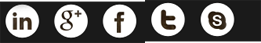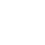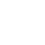18929295505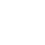2324774509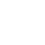admin@dgyouchen.com